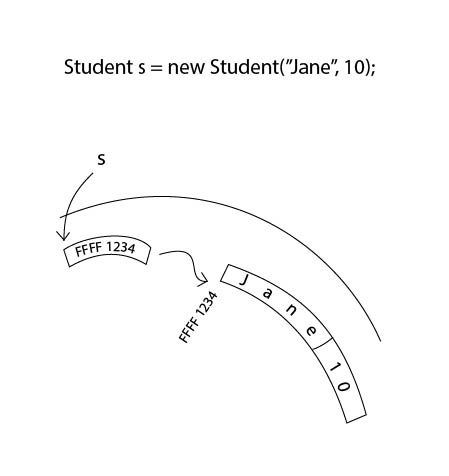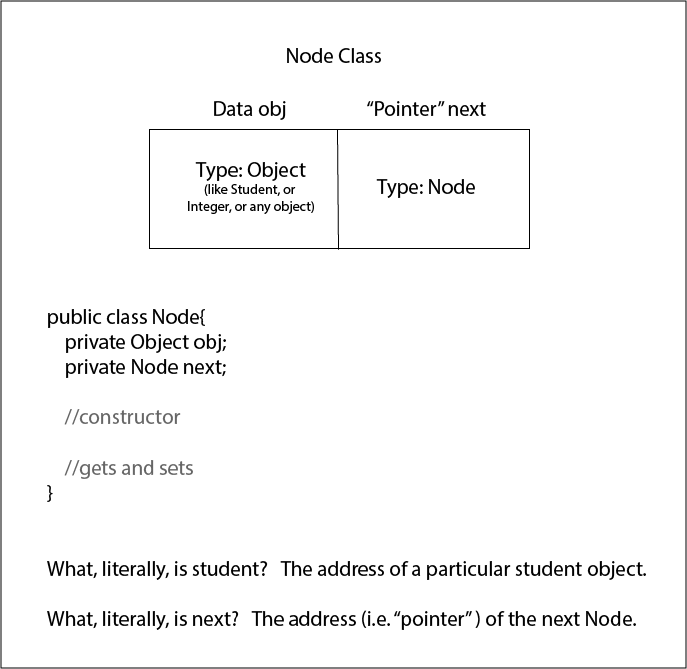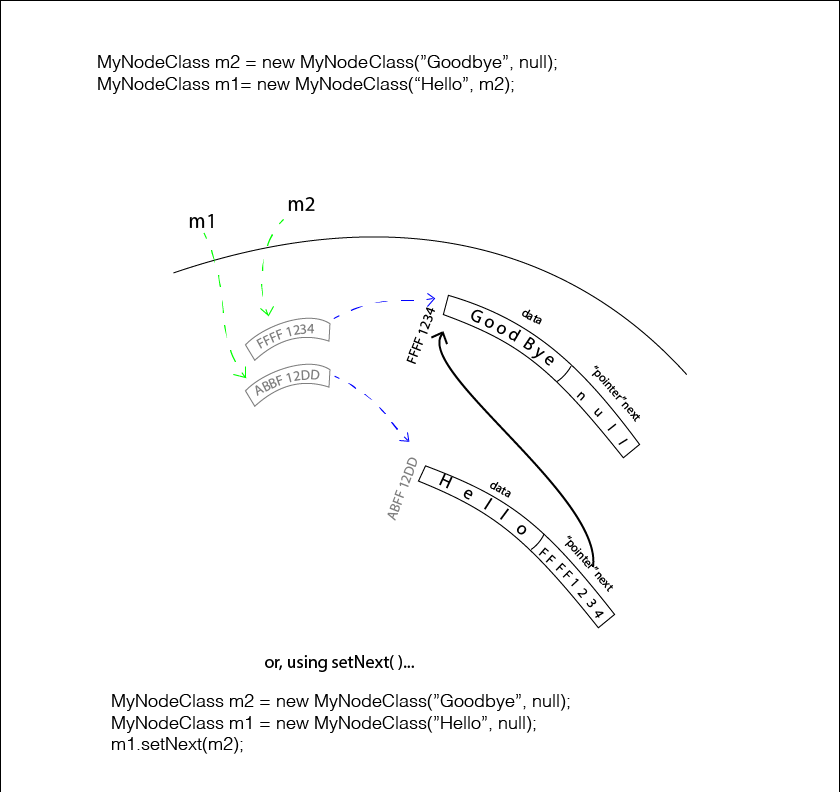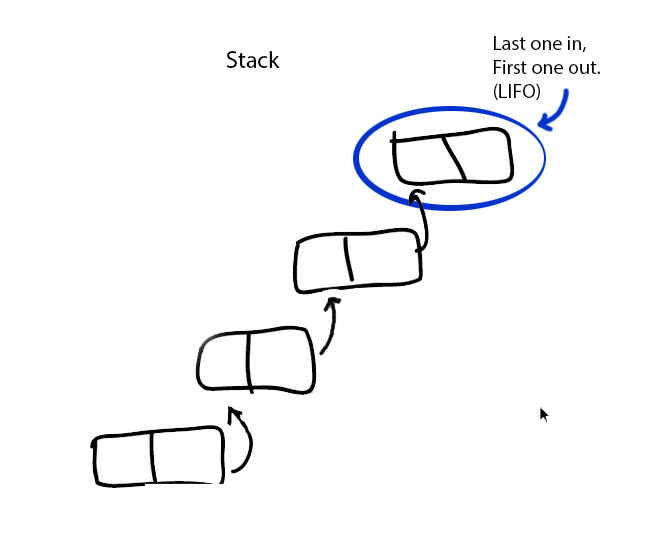Logout

D.4.5

Define the term object reference.

Teaching Note:

As typified by simple classes that are self-referential.

Sample Question:

sdfsdfsf

JSR Notes:

There are two things in this assessment statement. "object reference", which should be a review, and "self-referential classes", the new, powerful usage of object references.

### Review of Object Reference

First there's the same old same old very important concept of an object actually being, at its core, a memory address reference.

So:

Student s = new Student("Jane", 10);

makes a "reference" variable, which is actually/technically the address of where the Student object resides.

What is s technically/literally? s is a memory address, which points to the data associated with it. So yes, via dot notation (.getName( ) and .getGrade( )) we can access the data associated with s. And, yes, some place in memory, there is a String with the value of "Jane", and right beside it is an int, with the value of 10. But it's the address of where those two things reside which s actually is, so let's say FFFF1234.

or put another way:

So what is s, technically/literally? FFFF1234.

And/but what does s therefore represent; what useful information does it point to, that, using dot notation we can get at? The answer is the data residing at memory address FFFF1234, which is "Jane" and 10.So that's it for the review of what objects are, now onto the new concept which makes use of object references, self-referential classes.

-------------------------- " --------------------------

### Self-referential Classes

This is the kind of class in which at least one of its attributes is another instance of that class.

Here's a very simple example:

```public class Node( ){
Object obj = null; //so, since Object, can be any non-primitive thing
Node next = null;

public Node( ){
}

public Node(Object obj, Node next){
this.s = s;
this.next = next;
}
//sets
//gets
}```

Above, we can classify the class as being a self-referential class, because one of its attributes is of the type that it is; specifically, the MyClass class has attribute of type MyClass.

Here is how we diagram such a class, with it's two attributes:### The Utility of Self-referential Classes

So why would we want self-referential classes? With the use of self-referential classes, these kind of objects can easily find, and link to other objects of the same type, and thus form lists of those objects. This is what is done with the abstract data type (ADT) LinkedList, and others similar to it, like binary search trees.### Lists can thus be made

Lists are data structures in which instances of a certain type can link up to form an abstract data structure. This structure need not be made of instances which are physically side by side in memory, as is the case with arrays. Rather, through knowing where each next "link in the chain" is, in terms of memory, the full list can be navigated back and forth. The advantage of a list over an array is thus that the instances of the list can be scattered throughout memory; lists do not require one big contiguous memory space, as arrays do.### Summary

To understand how linking is possible, firstly keep in mind that objects are technically/literally themselves a 32 or 64 bit address. In a linked list, a Node does not just contain useful information like student name and grade, but also a "reference", or "pointer" Node to the next Node instance. And thus, step-by-step, link-by-link, these pointer links form lists.

See the next assessment statement for details of how this looks in practice.

(Final Note: Self-referential Class vs. Recursion

Don't confuse self-referential classes with recursion, if you have looked at recursion to this point. The two are similar in a way, but different, and each is used for a different purpose. With recursion, it is a method which calls another instance of itself, and this is done for the purposes of iteration. With self-referential classes, it is the class itself which has an instance that is of the same type of itself, and this is done for purposed of being able to locate another instance of the same class, usually as part of a list.)